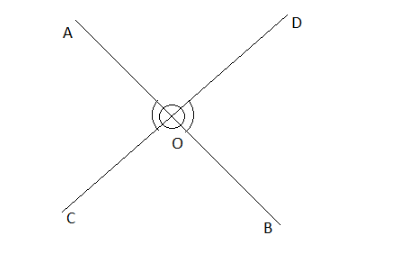Fill in the blanks:________ pair of vertically opposite angle are formed by the intersection of $2$ lines.Verified
119.7k+ views
Hint: First of all we will draw the intersection of two lines and then will find the pair of angles. Always remember that pair means group of two. So when we intersect two lines we get four angles and then we will make a pair of vertically opposite angles and then will count accordingly.